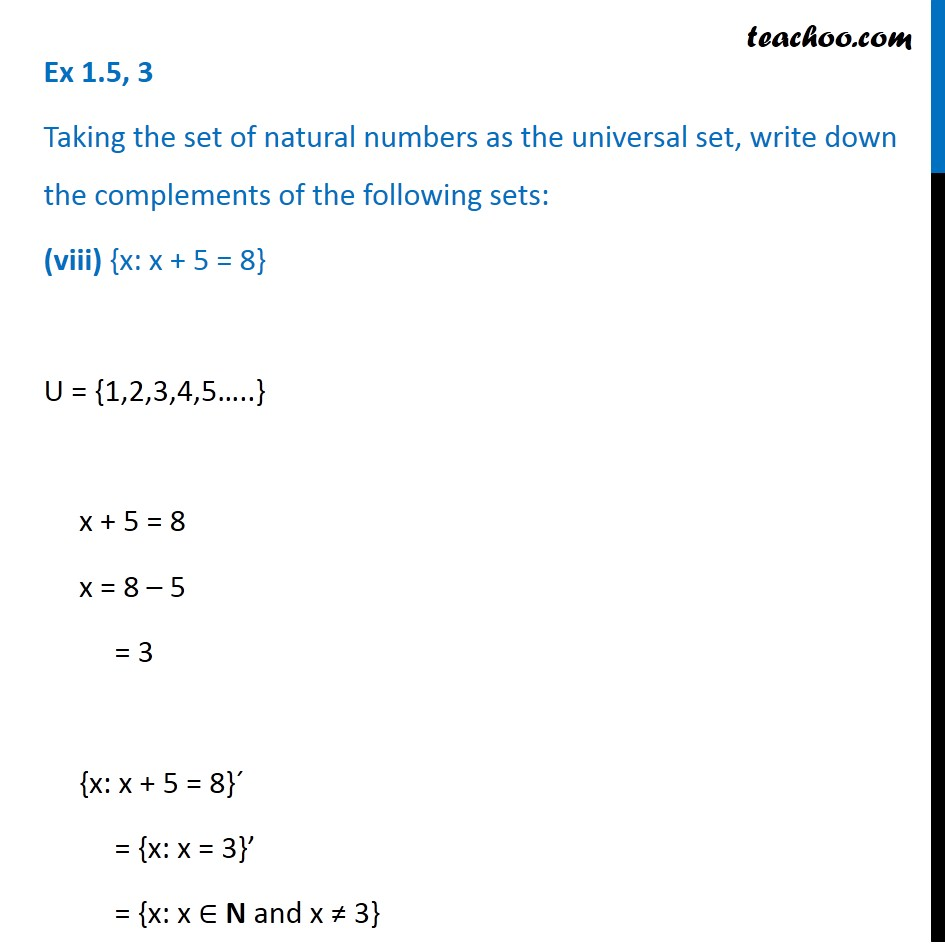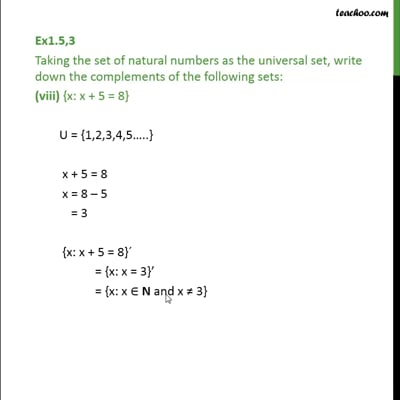Ex 1.5

Chapter 1 Class 11 Sets
Serial order wiseThis video is only available for Teachoo black users

Get live Maths 1-on-1 Classs - Class 6 to 12

### Transcript

Ex 1.5, 3 Taking the set of natural numbers as the universal set, write down the complements of the following sets: (viii) {x: x + 5 = 8} U = {1,2,3,4,5…..} x + 5 = 8 x = 8 – 5 = 3 {x: x + 5 = 8}´ = {x: x = 3}’ = {x: x ∈ N and x ≠ 3}